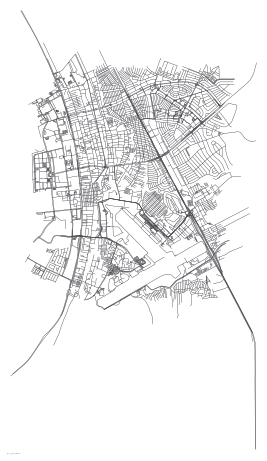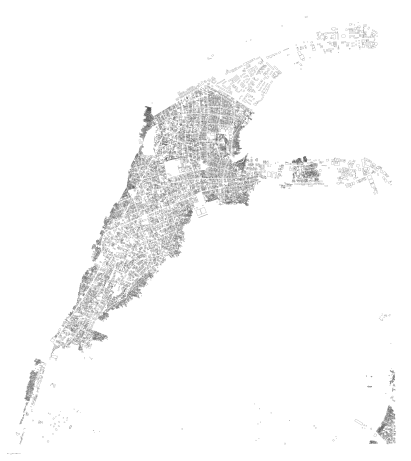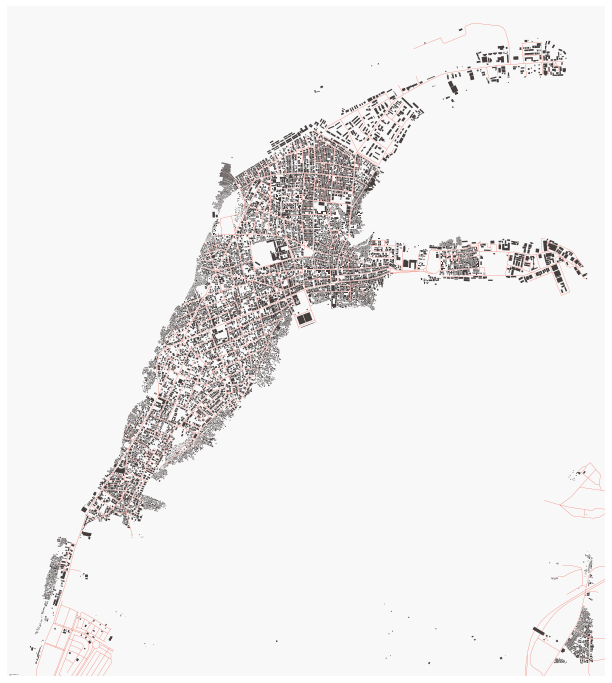# Working with Shapefiles and OSM Part II of II¶

Author: Erika Fille Legara

You are free to use (or change) this notebook for any purpose you'd like. However, please respect the MIT License that governs its use, and for copying permission.

## Description¶

This recipe is the second of a series of Python notebooks on shapefiles and OSM files that I am working on. After going through the notebook, you should be able to plot maps as in the images below.First things first, import the necessary packages. The Basemap package is used for drawing and plotting maps (and also for reading shapefiles). The shapefile package provides read and write support for the ESRI Shapefile format.

In :
try:
from mpl_toolkits.basemap import Basemap
from matplotlib.patches import Polygon
from matplotlib.collections import PatchCollection
from matplotlib.patches import PathPatch
import shapefile
import os.path
import matplotlib.pyplot as plt
import urllib
%matplotlib inline
import numpy as np
except:
import traceback
traceback.print_exc()
raise ImportError('Something failed, see above.')


The shapefile used here (for the Philippines and its municipalities) is obtained from GADM, which provides other shapefiles for other countries. The next code cell is only necessary to obtain the boundaries of a given administrative region. If you already have the boundary of your region of interest, you may skip the next three code cells.

In :
phl2 = shapefile.Reader("PHL_adm_shp/PHL_adm2")


## Fields (Records) + Geometries¶

Now, let's pull out the shapes and records and obtain the bounding-box for each city. I just want to reiterate that the code cell below is not necessary if you already have a bounding-box in mind.

In :
citiesRecs = phl2.shapeRecords()
cities_bbox = {}

for entry in citiesRecs:
cities_bbox[entry.record] = entry.shape.bbox

cities_bbox.items()[0:5]

Out:
[('Tagana-An',
[125.52970123291061, 9.65027046203636, 125.70527648925791, 9.769089698791568]),
('Talitay',
[124.32147979736328, 6.983870029449747, 124.41300964355484, 7.08224010467552]),
('Dumalneg',
[120.79208374023438, 18.415330886840877, 120.88435363769545, 18.531850814819375]),
('Aloguinsan',
[123.5244369506836, 10.143600463867188, 123.63102722167966, 10.248220443725543]),
('Bongabong',
[121.24404144287121, 12.618599891662598, 121.55500030517587, 12.798540115356673])]

We can see from the previous cell that for each city we have the coordinates of their bounding-box. For example, for "Tagana-An", the lower-left corner of the bounding-box is at latitude and longitude 9.65027046203636 and 125.52970123291061, respectively; while the upper-right corner has (9.769089698791568, 125.70527648925791).

The first 20 cities in the shapefile are listed below.

In :
print cities_bbox.keys()[0:20]

['Tagana-An', 'Talitay', 'Dumalneg', 'Aloguinsan', 'Bongabong', 'Villaviciosa', 'Solsona', 'Infanta', 'Malabon', 'Barobo', 'Minalin', 'Caramoran', 'San Jacinto', 'Mallig', 'Santa Ignacia', 'Tabuk City', 'Pandi', 'Tongkil', 'General Macarthur', 'General Emilio Aguinaldo']


## OpenStreetMap¶

In :
getfile = urllib.URLopener()


Let's get the OSM data for each of the cities in Metro Manila.

In :
metro_manila = ["Manila", "Quezon City", "Pateros", "Kalookan City", "Las Piñas", "Makati City",
"Malabon", "Mandaluyong", "Marikina", "Muntinlupa", "Navotas", "Parañaque", "Pasay City",
"Pasig City", "Taguig", "Valenzuela"]


I use XAPI to download city information. According to OSM's wiki page:

XAPI and Overpass API allow to download custom data sets like arbitrary bounding boxes, elements with specific tags, public transport networks or other features. [...] The API is limited to bounding boxes of about 0.5 degree by 0.5 degree and you should avoid using it if possible. For larger areas you might try to use XAPI [...]

IMPORTANT NOTE: If the code chunk results to a 504 error, the issue is on the server side (OSM overpass); not the code. You may have to wait to download again.

If a query is rejected due to too much resource consumption, this is now answered with HTTP status code 504

In :
urlhead = "http://overpass.osm.rambler.ru/cgi/xapi_meta?*[bbox="
urltail = "]"

for k in metro_manila:
url = urlhead + str(cities_bbox[k]) + "," + str(cities_bbox[k]) + "," + \
str(cities_bbox[k]) + "," + str(cities_bbox[k]) + urltail

if not os.path.exists("./cities_osm/"):
print "Creating cities_osm directory..."
os.makedirs("./cities_osm/")

fname = "./cities_osm/"+ str(k)+".osm"

if not os.path.isfile(fname):
try:
getfile.retrieve(url, fname)
except IOError as err:
print "Whoops! Looks like the server is busy... consuming too much resource. Let's wait a bit."
print "Error: ", err
break


We now have the OSM files for the cities we are interested in. Let's see what is inside an OSM file. Let's have a look at the first few lines of one OSM file.

In :
f = open("./cities_osm/Pasay City.osm",'rb')
print line.strip("\n")
f.close()

<?xml version="1.0" encoding="UTF-8"?>
<osm version="0.6" generator="Overpass API">
<note>The data included in this document is from www.openstreetmap.org. The data is made available under ODbL.</note>
<meta osm_base="2016-07-30T04:25:02Z"/>


Above, we are looking at the header of the file. The OSM files we have are XML files. I am not going to elaborate on this one, but if you want to learn more about the file formatting, please follow this link.

The major tools in the OSM universe use an XML format following a XML schema definition that was first used by the API only. Basically it is a list of instances of our data primitives (nodes, ways, and relations).

To read more about the different elements of an OSM file (or the data primitives), follow this link. As summarized in the site:

Elements are the basic components of OpenStreetMap's conceptual data model of the physical world. They consist of

• nodes (defining points in space),
• ways (defining linear features and area boundaries), and
• relations (which are sometimes used to explain how other elements work together).

All of the above can have one or more associated tags (which describe the meaning of a particular element).

In this recipe, we are more interested in the nodes and the ways. Below, we show how a node element looks and how a way element looks.

#### Node Element¶

In :
f = open("./cities_osm/Pasay City.osm",'rb')
print line.strip("\n")
f.close()

  <node id="2408221587" lat="14.5165490" lon="120.9914944" version="2" timestamp="2013-08-06T08:57:48Z" changeset="17238376" uid="81841" user="schadow1">
<tag k="name" v="Bay 9 Aguinaldo"/>
<tag k="tourism" v="information"/>
</node>
<node id="2408221588" lat="14.5169370" lon="120.9909399" version="2" timestamp="2013-08-06T08:57:48Z" changeset="17238376" uid="81841" user="schadow1">
<tag k="amenity" v="place_of_worship"/>
<tag k="denomination" v="catholic"/>
<tag k="name" v="Chapel"/>
<tag k="religion" v="christian"/>
</node>


For the previous code chunk, we can see that the first node element has the following info: id, lat, lon, version, timestamp, changeset, uid, user. The same element also has the following tags: name, source, and tourism with corresponding values "Bay 9 Aguinaldo", "Schadow1 Expeditions", and "information". The next node with id="2408221588" has more tags than the first one. These tags are helpful in identifying amenities, among others. Node with id="2408221588" is an "amenity"; and, in particular, it is a "place_of_worship".

#### Way Element¶

Below, we see that the way element have nd "children"---these are the nodes associated to that particular way. Using the nd reference, we can lookup the node information for a given ID (reference). And, as is above, the elements have attributes and tags. The second way element, for example, with id="387706704", is tagged as a highway; in particular, it offers a service (a drive-through). The element is also tagged as oneway.

In :
f = open("./cities_osm/Pasay City.osm",'rb')
print line.strip("\n")
f.close()

  <way id="387706703" version="1" timestamp="2015-12-25T18:09:16Z" changeset="36163163" uid="11126" user="seav">
<nd ref="3909506583"/>
<nd ref="3909506582"/>
<nd ref="3909506581"/>
<nd ref="3909506580"/>
<nd ref="3909506578"/>
<nd ref="3909506579"/>
<nd ref="3909506584"/>
<nd ref="3909506585"/>
<nd ref="3909506586"/>
<nd ref="3909506587"/>
<nd ref="3909506588"/>
<nd ref="3909506589"/>
<tag k="highway" v="service"/>
</way>
<way id="387706704" version="1" timestamp="2015-12-25T18:09:16Z" changeset="36163163" uid="11126" user="seav">
<nd ref="3909506593"/>
<nd ref="3909506613"/>
<nd ref="3909506615"/>
<nd ref="3909506616"/>
<nd ref="3909506618"/>
<nd ref="3909506622"/>
<nd ref="3909506620"/>
<nd ref="3909506617"/>
<nd ref="3909506611"/>
<nd ref="3909506610"/>
<nd ref="3909506607"/>
<nd ref="3909506595"/>
<tag k="highway" v="service"/>
<tag k="oneway" v="yes"/>
<tag k="service" v="drive-through"/>
</way>
<way id="387706705" version="1" timestamp="2015-12-25T18:09:16Z" changeset="36163163" uid="11126" user="seav">
<nd ref="3909506590"/>
<nd ref="3909506594"/>
<nd ref="3909506597"/>
<nd ref="3909506601"/>
<tag k="oneway" v="yes"/>
</way>


### Manipulating OSM elements and tags¶

To easily manipulate the OSM files, I use the Python package osmread. It is a simple library for reading the files; it allows us to parse a file and choose (or filter) specific element types.

In :
from osmread import parse_file, Way, Node, Relation


For example, if I want to obtain all the highways in the file, I need to track all Way elements and look at their tags---whether or not they have the highway tag. Check the getHighways() function written below.

In :
def getHighWays(osmfile):
all_nodes = {}
way_nodes = []

for entity in parse_file(osmfile):
if isinstance(entity, Node):
all_nodes[entity.id] = [entity.lon, entity.lat]
if isinstance(entity, Way) and 'highway' in entity.tags:
way_nodes.append(list(entity.nodes))
return all_nodes, way_nodes


We are also taking note of all the nodes associated with a highway Way entity; remember the nd tag. We use this later for plotting. We implement the same process to look for buildings in the file. Since a building is coded with a set of nodes, we look for a Way element with the building tag. See the getBuildings() function below.

In :
def getBuildings(osmfile):
all_nodes = {}
way_nodes = []
tags = []
for entity in parse_file(osmfile):
if isinstance(entity, Node):
all_nodes[entity.id] = [entity.lon, entity.lat]
if isinstance(entity, Way) and "building" in entity.tags:
way_nodes.append(list(entity.nodes))
return all_nodes, way_nodes


### Going back to shapefiles¶

Now that we have the highway and building entities and the associated shape (lat, lon) information, we are ready to plot them. There may be other ways that exist out there, but I have opted to create individual shapefiles for these. For the roads, we write polylines (shapefile); for the buildings, polygons.

In :
def createPolyLine(all_nodes, way_nodes, region):
w = shapefile.Writer(shapefile.POLYLINE)

lon_list = []
lat_list = []

for way in way_nodes:
parts = []
for node in way:
lon = float(all_nodes[node])
lat = float(all_nodes[node])
parts.append([lon,lat])
lon_list.append(lon)
lat_list.append(lat)
w.poly(parts=[parts], shapeType=shapefile.POLYLINE)

w.field('ID')
w.field('SECOND_FLD') ## This is an arbitrary field

for i in range(len(way_nodes)):
w.record(i,'Line') ## This an arbitrary information

w.save(region)

return lon_list, lat_list

In :
def createPolygon(all_nodes, way_nodes, region):
w = shapefile.Writer(shapefile.POLYGON)

lon_list = []
lat_list = []

for way in way_nodes[:-1]:
parts = []
for node in way:
lon = float(all_nodes[node])
lat = float(all_nodes[node])
parts.append([lon,lat])
lon_list.append(lon)
lat_list.append(lat)
w.poly(parts=[parts])

w.field('ID')
w.field('SECOND_FLD') ## This is an arbitrary field
for i in range(len(way_nodes)):
w.record(i,'Polygon') ## This an arbitrary information

w.save(region)
return lon_list, lat_list


Using Pasay City as example, we will be generating the following files: [Pasay City line.dbf, Pasay City line.shp, Pasay City line.shx, Pasay City poly.dbf, Pasay City poly.shp, Pasay City line.shx]. Using the shapefiles, we can now plot the buildings and road networks using Basemap.

In :
def plotRoadNetwork(lon_list, lat_list, whichLine, fname):
_ = plt.clf()
_ = plt.figure(figsize=(8,8))
m = Basemap(llcrnrlon=min(lon_list)-0.001,
llcrnrlat=min(lat_list)-0.001,
urcrnrlon=max(lon_list)+0.001,
urcrnrlat=max(lat_list)+0.001,
resolution='i', projection='tmerc', lat_0 = np.mean(lat_list), lon_0 = np.mean(lon_list))
_ = m.readshapefile(whichLine,whichLine, color="#0f0f13", zorder=2, linewidth=0.3)
_ = m.drawmapboundary(color='white',  fill_color="#ffffff", linewidth=1)
_ = plt.text(1,2,"by @eflegara", fontsize=2, color="gray")
plt.show()
#_ = plt.savefig("./maps/" +fname, dpi=300, bbox_inches="tight")
#_ = plt.clf()

In :
region = "Pasay City"

all_nodes, way_nodes = getHighWays("./cities_osm/" + str(region) + ".osm")
lon_list, lat_list = createPolyLine(all_nodes, way_nodes, region + " line")
_ = plotRoadNetwork( lon_list, lat_list, region + " line", region + "-line"+".png")

<matplotlib.figure.Figure at 0x115db8350>In :
def plotBuildings(lon_list, lat_list, whichPoly, fname):
_ = plt.clf()
_ = plt.figure(figsize=(8,8))
m = Basemap(llcrnrlon=min(lon_list)-0.001,
llcrnrlat=min(lat_list)-0.001,
urcrnrlon=max(lon_list)+0.001,
urcrnrlat=max(lat_list)+0.001,
resolution='i', projection='tmerc', lat_0 = np.mean(lat_list), lon_0 = np.mean(lon_list))
_ = m.readshapefile(whichPoly, whichPoly, color="#000000", zorder=2, linewidth=0.1)
_ = m.drawmapboundary(color='white',  fill_color="#ffffff", linewidth=1)
_ = m.fillcontinents(color='#ffffff', lake_color ="#ffffff")
_ = plt.text(1,2,"by @eflegara", fontsize=2, color="gray")
_ = plt.show()
#_ = plt.savefig("./buildings/" + fname, dpi=900, bbox_inches="tight")
#_ = plt.clf()

In :
region = "Cavite City"

all_nodes, way_nodes = getBuildings("./cities_osm/" + str(region) + ".osm")
lon_list, lat_list = createPolygon(all_nodes, way_nodes, region + " poly")
_ = plotBuildings( lon_list, lat_list, region + " poly", region + "-poly"+".png")

<matplotlib.figure.Figure at 0x108ce5d10>### Together¶

In :
interests = ["Cavite City", "Manila", "Taguig", "Makati City", "Iloilo City",
"Pasig City", "Kalookan City", "Mandaluyong", "Pasay City",
"Baguio City", "Cagayan de Oro City", "Quezon City", "Navotas", "Parañaque",
"Valenzuela"]

for region in interests[0:1]:
_ = plt.clf()
_ = plt.figure( figsize=(12,12))
m = Basemap(llcrnrlon = cities_bbox[region]-0.001,
llcrnrlat = cities_bbox[region]-0.001,
urcrnrlon = cities_bbox[region]+0.001,
urcrnrlat = cities_bbox[region]+0.001,
resolution='i', projection='tmerc',
lat_0 = np.mean([cities_bbox[region],cities_bbox[region]]),
lon_0 = np.mean([cities_bbox[region],cities_bbox[region]]))

m.drawmapboundary(fill_color='#F8F8F8', linewidth=0)
m.fillcontinents(color='#F8F8F8',lake_color='#F8F8F8')
m.drawcoastlines(color="#ffffff", linewidth=0.01)

m.readshapefile("./city_shapefiles/" + str(region) + " poly", "region_poly",
drawbounds=False)
m.readshapefile("./city_shapefiles/" + str(region) + " line", "region_line",
color="#EF2E18", zorder=2, linewidth=0.2)
patches   = []
ax = plt.gca()
for info, shape in zip(m.region_poly_info, m.region_poly):
#print info
patches.append( Polygon(np.array(shape), True) )


<matplotlib.figure.Figure at 0x112883e50>Some of the city maps I have generated using the code above.